JEE  >  Physics Test 4 - Electrostatics, Capacitance, Current Electricity

# Physics Test 4 - Electrostatics, Capacitance, Current Electricity

Test Description

## 25 Questions MCQ Test JEE Main & Advanced Mock Test Series | Physics Test 4 - Electrostatics, Capacitance, Current Electricity

Physics Test 4 - Electrostatics, Capacitance, Current Electricity for JEE 2023 is part of JEE Main & Advanced Mock Test Series preparation. The Physics Test 4 - Electrostatics, Capacitance, Current Electricity questions and answers have been prepared according to the JEE exam syllabus.The Physics Test 4 - Electrostatics, Capacitance, Current Electricity MCQs are made for JEE 2023 Exam. Find important definitions, questions, notes, meanings, examples, exercises, MCQs and online tests for Physics Test 4 - Electrostatics, Capacitance, Current Electricity below.
Solutions of Physics Test 4 - Electrostatics, Capacitance, Current Electricity questions in English are available as part of our JEE Main & Advanced Mock Test Series for JEE & Physics Test 4 - Electrostatics, Capacitance, Current Electricity solutions in Hindi for JEE Main & Advanced Mock Test Series course. Download more important topics, notes, lectures and mock test series for JEE Exam by signing up for free. Attempt Physics Test 4 - Electrostatics, Capacitance, Current Electricity | 25 questions in 60 minutes | Mock test for JEE preparation | Free important questions MCQ to study JEE Main & Advanced Mock Test Series for JEE Exam | Download free PDF with solutions
 1 Crore+ students have signed up on EduRev. Have you?
Physics Test 4 - Electrostatics, Capacitance, Current Electricity - Question 1

### Figure shows some of the electric field lines corresponding to an electric field. The figure suggests that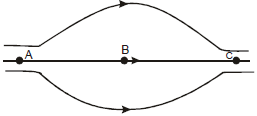Detailed Solution for Physics Test 4 - Electrostatics, Capacitance, Current Electricity - Question 1

At point A and C, electric field lines are dense and equally spaced, so EA = EC
While at B they are far apart.
∴ EA = E> EB

Physics Test 4 - Electrostatics, Capacitance, Current Electricity - Question 2

### When the separation between two charges is increased, the electric potential energy of the charges

Detailed Solution for Physics Test 4 - Electrostatics, Capacitance, Current Electricity - Question 2

Potential  energy between two charges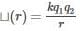Now if r increases and q1 and q2 are of same sign then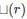decrases. if q1 and q2 are of oppositeincreases.
Therefore depending on signs of q1 and qmay increase or decrease.

Physics Test 4 - Electrostatics, Capacitance, Current Electricity - Question 3

### An electric dipole is placed in a uniform electric field. The net electric force on the dipole

Detailed Solution for Physics Test 4 - Electrostatics, Capacitance, Current Electricity - Question 3 As dipole is combination of equal positive and negative charges, when an electric field is generated in the region of the dipole, torque can act on the dipole, but there will be no force on the dipole.
Physics Test 4 - Electrostatics, Capacitance, Current Electricity - Question 4
Electric charge are distributed in a small volume. The flux of the electric field through a spherical surface of radius 10cm surrounding the total charge is 25V-m The flux over a concentric sphere of radius 20cm will be
Physics Test 4 - Electrostatics, Capacitance, Current Electricity - Question 5

A charge q is placed at the centre of the open end of a cylindrical vessel in figure. The flux of the electric field through the surface of the vessel is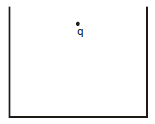Detailed Solution for Physics Test 4 - Electrostatics, Capacitance, Current Electricity - Question 5

when a charge Q is placed at the centre of the open end of a cylindrical vessel then only half of the charge will contribute to the flux,because half will lie inside the surface and half will outside the surface so flux=Q/2ε0

Physics Test 4 - Electrostatics, Capacitance, Current Electricity - Question 6
Mark the correct option
Physics Test 4 - Electrostatics, Capacitance, Current Electricity - Question 7

Two capacitors each having capacitance C and breakdown voltage V are joined in series. The capacitance and the breakdown voltage of the combination will be

Detailed Solution for Physics Test 4 - Electrostatics, Capacitance, Current Electricity - Question 7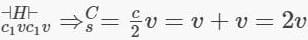Physics Test 4 - Electrostatics, Capacitance, Current Electricity - Question 8

A dielectric slab is inserted between the plates of an isolated capacitor. The force between the plates will

Detailed Solution for Physics Test 4 - Electrostatics, Capacitance, Current Electricity - Question 8
Here,the capacitor is isolated. It is already charged or else there would be no force. But when the dielectric is inserted, the charge remains fixed. If the capacitor were connected to a voltage source, then current would flow to keep the voltage fixed and then the force would increase.The voltage goes down even though there is no current in or out.The permittivity of any material is greater than the permittivity of free space. Therefore,When we insert the dielectric slab the capacitance rises.

Physics Test 4 - Electrostatics, Capacitance, Current Electricity - Question 9

Two metal spheres of capacitances C1 & C2   carry some charges. They are put in contact and then separated. The final charges Q1  and Q2  on them will satisfy

Physics Test 4 - Electrostatics, Capacitance, Current Electricity - Question 10

Two resistances R and 2R are connected in parallel in an electric circuit. The thermal energy developed in R and 2 R are in the ratio

Detailed Solution for Physics Test 4 - Electrostatics, Capacitance, Current Electricity - Question 10

Thermal energy = V2t/R
In parellel arrangement V is constant
So, for a time t
Thermal energy ∝ 1/R
Ratio of their thermal energy = R₂ : R₁ = 2R : R = 2 : 1

Physics Test 4 - Electrostatics, Capacitance, Current Electricity - Question 11
A uniform wire of resistance 50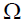is cut into 5 equal parts. These parts are now connected in parallel. The equivalent resistance of the combination is
Detailed Solution for Physics Test 4 - Electrostatics, Capacitance, Current Electricity - Question 11 Each part resistance is 10 ohm as R is directly proportional to length now 10 ohms 5 resistance are connected in parallel. Therefore 2 is equivalent resistance.
Physics Test 4 - Electrostatics, Capacitance, Current Electricity - Question 12
The net resistance of an ammeter should be small to ensure that
Detailed Solution for Physics Test 4 - Electrostatics, Capacitance, Current Electricity - Question 12 Based on basic construction and implementation of ammeter,it is in connected in series in a circuit and should not appreciably change the current to be measured.
Physics Test 4 - Electrostatics, Capacitance, Current Electricity - Question 13

The equivalent capacitance between the points A and B of five identical capacitors each of capacity C, will be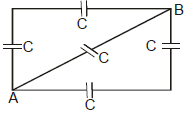Detailed Solution for Physics Test 4 - Electrostatics, Capacitance, Current Electricity - Question 13 The two identical branches capacitors have capacitance equal to C/2 each.
adding these in parallel will give you C/2 +C/2+C = 2C.
Physics Test 4 - Electrostatics, Capacitance, Current Electricity - Question 14

The electric field intensity at a point situated 4 meters from a point charge is 200 N/C. If the distance is reduced to 2 meters, the field intensity will be

Physics Test 4 - Electrostatics, Capacitance, Current Electricity - Question 15

If an electron is brought towards an another electron, the electric potential energy of the system

Physics Test 4 - Electrostatics, Capacitance, Current Electricity - Question 16
Two identical conductors of a copper and aluminium are placed in an identical electric field. What is the magnitude of induced charge in the aluminium ?
Detailed Solution for Physics Test 4 - Electrostatics, Capacitance, Current Electricity - Question 16 As aluminum and copper are metals, their mobile electrons move under the influence of the external field until they reach the surface of the metal and collect there. External electric fields induce surface charges on metal objects that exactly cancel the field within. Since the field applied is same in both case, therefore induced charge will be the same. Since both are metals so equal amount of charge will induce on them.
Physics Test 4 - Electrostatics, Capacitance, Current Electricity - Question 17

The electric field intensity at a point situated 4 meters from a point charge is 200 N/C. If the distance is reduced to 2 meters, the field intensity will be

Detailed Solution for Physics Test 4 - Electrostatics, Capacitance, Current Electricity - Question 17 200=KQ/16-------equ-1
E=KQ/4-------------equ-2
on dividing1by 2
E= 800
Physics Test 4 - Electrostatics, Capacitance, Current Electricity - Question 18

An electric dipole is placed at an angle of 30° with an electric field intensity 2 × 105 N/C. It experiences a torque equal to 4 N m. The charge on the dipole, if the dipole length is 2 cm, is

Physics Test 4 - Electrostatics, Capacitance, Current Electricity - Question 19

Shown in the figure is a distribution of charges. The flux of electric field due to these charges through the surface S is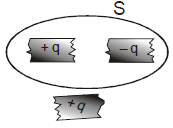Detailed Solution for Physics Test 4 - Electrostatics, Capacitance, Current Electricity - Question 19

Flux flowing through a body depends on the net charge enclosed inside the surface, here net charge inside is zero so flux is also zero. And the third charge is outside so it will not effect the flux.

Physics Test 4 - Electrostatics, Capacitance, Current Electricity - Question 20

Ten identical cells each of emf E and internal resistance r are connected in series to form a closed circuit. An ideal voltmeter connected across the three cells will read

Detailed Solution for Physics Test 4 - Electrostatics, Capacitance, Current Electricity - Question 20

No. of cells = 10
Emf = E
Internal resistance = r
Let I = current through the ckt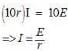Now potential (V) of across each cell will be
V = E -Ir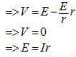We see there being no load resistance internal resistance play no effect on the observed emf
Thus the pd across 3 cells will be
E + E + E = 3Ir = 3E

*Answer can only contain numeric values
Physics Test 4 - Electrostatics, Capacitance, Current Electricity - Question 21

A dipole consists of two particles one with charge +1µC and mass 1kg and the other with charge –1µC and mass 2kg separated by a distance of 3m. for small oscillations about its equilibrium position, the angular frequency, when placed in a uniform electric field of 20kV/m is :-

Detailed Solution for Physics Test 4 - Electrostatics, Capacitance, Current Electricity - Question 21

Restoring torque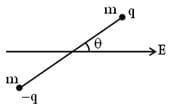τ = – PE sin θ
τ = – PE θ, for small θ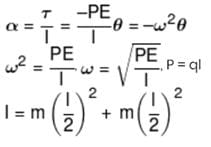*Answer can only contain numeric values
Physics Test 4 - Electrostatics, Capacitance, Current Electricity - Question 22

A solid conducting sphere having a charge Q is surrounded by an uncharged concentric conducting hollow spherical shell. Let the potential difference between the surface of the solid sphere and that of the outer surface of the hollow shell be V. If the shell is now given a charge –3Q, the new potential difference between the same two surface is xV, then x is :-

Detailed Solution for Physics Test 4 - Electrostatics, Capacitance, Current Electricity - Question 22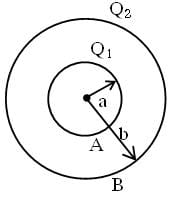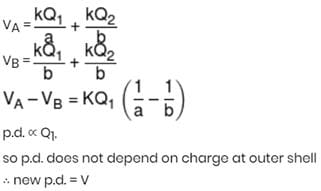*Answer can only contain numeric values
Physics Test 4 - Electrostatics, Capacitance, Current Electricity - Question 23

A potentiometer is used for the comparison of e.m.f. of two cells E1 and E2. For cell E1 the no deflection point is obtained at 20 cm and for E2 the no deflection point is obtained at 30 cm. The ratio of their e.m.f.’s is N/3 then N is:

Detailed Solution for Physics Test 4 - Electrostatics, Capacitance, Current Electricity - Question 23

Ratio will be equal to the ratio of no deflection lengths i.e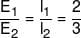*Answer can only contain numeric values
Physics Test 4 - Electrostatics, Capacitance, Current Electricity - Question 24

The equivalent resistance of the arrangement of resistances shown in adjoining figure between the points A and B is N × 4 ohm then N is: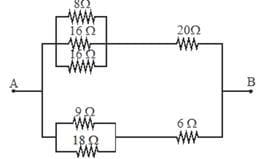Detailed Solution for Physics Test 4 - Electrostatics, Capacitance, Current Electricity - Question 24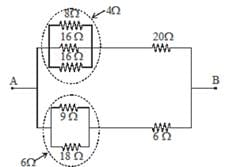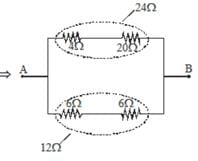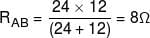*Answer can only contain numeric values
Physics Test 4 - Electrostatics, Capacitance, Current Electricity - Question 25

In the circuit shown here C1 = 6μ F, C2 = 3μ F and battery B = 20V. The switch sis first closed. It is then opened and afterwards sis closed. What is the charge finally on C2.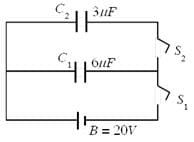## JEE Main & Advanced Mock Test Series

2 videos|325 docs|189 tests
 Use Code STAYHOME200 and get INR 200 additional OFF Use Coupon Code
Information about Physics Test 4 - Electrostatics, Capacitance, Current Electricity Page
In this test you can find the Exam questions for Physics Test 4 - Electrostatics, Capacitance, Current Electricity solved & explained in the simplest way possible. Besides giving Questions and answers for Physics Test 4 - Electrostatics, Capacitance, Current Electricity, EduRev gives you an ample number of Online tests for practice

## JEE Main & Advanced Mock Test Series

2 videos|325 docs|189 tests